• report
Disclaimer
Click for Disclaimer
This Post is over a year old (first published about 3 years ago). As such, please keep in mind that some of the information may no longer be accurate, best practice, or a reflection of how I would approach the same thing today.
• infoFull Post Details
info_outlineClick for Full Post Details
Date Posted:
Jan. 31, 2019
Last Updated:
Jan. 27, 2021
• classTags
classClick for Tags
Tags:

I’m used to Google Sheets having built-in formulae for practically everything, so I was surprised when I needed to Base64 encode a string today and could not find a function to do so. The easiest solution is to use a “Custom Function“, which allows you write your own named function in JavaScript, and then call that function from anywhere in your Google Sheets file, just as if it was a built-in function. That is Solution A, below. Skip to Solution B if you want to see how I created a custom formula that only uses native Google Sheets functions!

## Solution A – Using a Google Sheets Script “Custom Function”:

This is super easy. Just add the following code to the scripts file attached to your Google Sheet, by going to “Menu > Tools > Script Editor”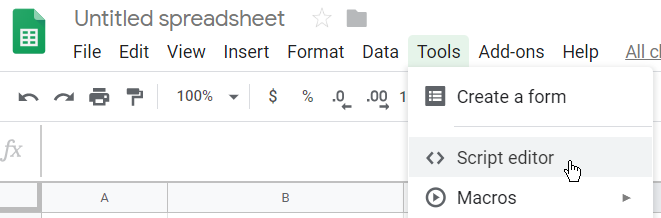``````
/**
* Base64 Encode Input
* @param {any | Array<any[]>} input - Input cell, or range of cells
* @param {boolean} [OPT_webSafe=true] - If should use websafe variant of base64
* @param {boolean} [OPT_plainText=false] - If should treat input as plaintext instead of UTF-8
*/
function base64Encode(input, OPT_webSafe, OPT_plainText) {
if (!input) return input;
const charSet = OPT_plainText ? Utilities.Charset.US_ASCII : Utilities.Charset.UTF_8;
const useWebSafe = OPT_webSafe !== false;
const encoder = useWebSafe ? Utilities.base64EncodeWebSafe : Utilities.base64Encode;
if (Array.isArray(input)) {
return input.map(t => base64Encode(t, OPT_webSafe, OPT_plainText));
}

return encoder(input, charSet); }
/**

Base64 Decode Input
@param {any | Array<any[]>} input - Input cell, or range of cells
@param {boolean} [OPT_webSafe=true] - If should use websafe variant of base64
@param {boolean} [OPT_plainText=false] - If should treat input as plaintext instead of UTF-8 */ function base64Decode(input, OPT_webSafe, OPT_plainText) { if (!input) return input; const charSet = OPT_plainText ? Utilities.Charset.US_ASCII : Utilities.Charset.UTF_8; const useWebSafe = OPT_webSafe !== false; const decoder = useWebSafe ? Utilities.base64DecodeWebSafe : Utilities.base64Decode; if (Array.isArray(input)) { return input.map(t => base64Decode(t, OPT_webSafe, OPT_plainText)); }

````return Utilities.newBlob(decoder(input, charSet)).getDataAsString(); } ````

Once you have pasted and saved that code into the script editor, switch back to your spreadsheet, and reload the page. You should now be able to use the custom function very easily. 99% of the time, you should be able to use it with default settings, like so: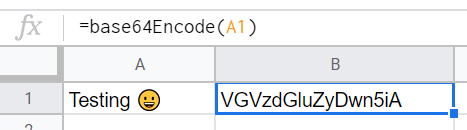You can even use the formula with an array of cells as the input,and it will automatically fill down!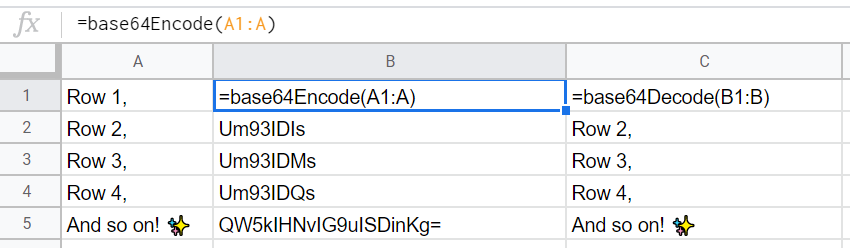### Breaking down the custom function:

This is basically the same code as above, but broken down into separate functions, so you can see how it works.

``````
function base64Encode(input) {
return Utilities.base64Encode(input,Utilities.Charset.UTF_8);
}
function base64EncodeWebSafe(input) {
return Utilities.base64EncodeWebSafe(input,Utilities.Charset.UTF_8);
}
function base64Decode(input) {
return Utilities.newBlob(Utilities.base64Decode(input,Utilities.Charset.UTF_8)).getDataAsString();
}
function base64DecodeWebSafe(input) {
return Utilities.newBlob(Utilities.base64DecodeWebSafe(input,Utilities.Charset.UTF_8)).getDataAsString();
}
``````

The main difference between WebSafe (aka “base64url”) and non-WebSafe is that “/” is replaced with “_” for WebSafe, since slashes are reserved in URLs for path separators. For more details see RFC-4648.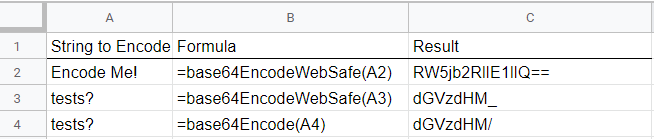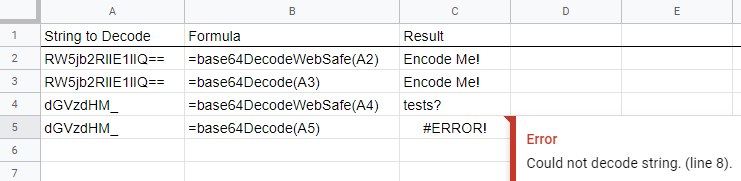Notice that the “could not decode string” error in the screenshot above is because you should not use base64DecodeWebSafe to decode something that was originally encoded with the WebSafe variant of Base64. In this case, it doesn’t like the “_” character, which is specific to the WebSafe variant. In general, you are probably safer just using the WebSafe version most of the time, if you want to avoid these kinds of errors.

EDIT: Thank you to Maxime for pointing out an error in my original code; I did not account for the fact that Utilities.base64Decode & Utilities.base64DecodeWebSafe both return byte-arrays, not strings. For some reason I only tested my encode functions and did not notice this. This post has been updated, and for further reference, see Maxime’s comments at the bottom, and this Apps Script doc page.

EDIT: I added the UTF-8 argument to all the functions, in case anyone is using this with non ASCII text (for example, a foreign language that uses accented or non Latin characters). If you are only using ASCII, you could leave off this argument, and Sheets will default. However, as ASCII is part of UTF-8, it doesn’t hurt to leave it.

## Solution B: “Pure Formula” function – No Google Apps Scripting required!

Custom functions through Google Sheets App Scripting is fast and simple. However, this is not always the most “performant” solution, and I felt like taking on a challenge today, so I actually came up with a formula that uses only native Google Sheets functions to Base64 encode a string – no scripting! This is also helpful if you are not allowed to use Scripts within your Google Sheets file due to restrictions placed by your employer, or you are just looking for the quickest copy and paste solution.

Here is the full (insanely long and complex) Google Sheets formula that converts text to Base64, nothing else required. Just replace “A2” with the cell you want to convert the text from:

``````
=CONCATENATE(JOIN("",ARRAYFORMULA(SWITCH(BIN2DEC(TEXT(SPLIT(REGEXREPLACE(CONCATENATE(JOIN("",ARRAYFORMULA(BASE(UNICODE(SPLIT(REGEXREPLACE(REGEXREPLACE(A2&"","(?s)(.{1})","\$1"&CHAR(127)),"'","''"),CHAR(127))),2,8))),REPT("0",(FLOOR((LEN(JOIN("",ARRAYFORMULA(BASE(UNICODE(SPLIT(REGEXREPLACE(REGEXREPLACE(A2&"","(?s)(.{1})","\$1"&CHAR(127)),"'","''"),CHAR(127))),2,8))))+(6-1))/6)*6)-LEN(JOIN("",ARRAYFORMULA(BASE(UNICODE(SPLIT(REGEXREPLACE(REGEXREPLACE(A2&"","(?s)(.{1})","\$1"&CHAR(127)),"'","''"),CHAR(127))),2,8)))))),"(.{6})","\$1/"),"/"),"000000")),0,"A",1,"B",2,"C",3,"D",4,"E",5,"F",6,"G",7,"H",8,"I",9,"J",10,"K",11,"L",12,"M",13,"N",14,"O",15,"P",16,"Q",17,"R",18,"S",19,"T",20,"U",21,"V",22,"W",23,"X",24,"Y",25,"Z",26,"a",27,"b",28,"c",29,"d",30,"e",31,"f",32,"g",33,"h",34,"i",35,"j",36,"k",37,"l",38,"m",39,"n",40,"o",41,"p",42,"q",43,"r",44,"s",45,"t",46,"u",47,"v",48,"w",49,"x",50,"y",51,"z",52,"0",53,"1",54,"2",55,"3",56,"4",57,"5",58,"6",59,"7",60,"8",61,"9",62,"-",63,"_",64,"="))),REPT("=",(FLOOR((LEN(JOIN("",ARRAYFORMULA(SWITCH(BIN2DEC(TEXT(SPLIT(REGEXREPLACE(CONCATENATE(JOIN("",ARRAYFORMULA(BASE(UNICODE(SPLIT(REGEXREPLACE(REGEXREPLACE(A2&"","(?s)(.{1})","\$1"&CHAR(127)),"'","''"),CHAR(127))),2,8))),REPT("0",(FLOOR((LEN(JOIN("",ARRAYFORMULA(BASE(UNICODE(SPLIT(REGEXREPLACE(REGEXREPLACE(A2&"","(?s)(.{1})","\$1"&CHAR(127)),"'","''"),CHAR(127))),2,8))))+(6-1))/6)*6)-LEN(JOIN("",ARRAYFORMULA(BASE(UNICODE(SPLIT(REGEXREPLACE(REGEXREPLACE(A2&"","(?s)(.{1})","\$1"&CHAR(127)),"'","''"),CHAR(127))),2,8)))))),"(.{6})","\$1/"),"/"),"000000")),0,"A",1,"B",2,"C",3,"D",4,"E",5,"F",6,"G",7,"H",8,"I",9,"J",10,"K",11,"L",12,"M",13,"N",14,"O",15,"P",16,"Q",17,"R",18,"S",19,"T",20,"U",21,"V",22,"W",23,"X",24,"Y",25,"Z",26,"a",27,"b",28,"c",29,"d",30,"e",31,"f",32,"g",33,"h",34,"i",35,"j",36,"k",37,"l",38,"m",39,"n",40,"o",41,"p",42,"q",43,"r",44,"s",45,"t",46,"u",47,"v",48,"w",49,"x",50,"y",51,"z",52,"0",53,"1",54,"2",55,"3",56,"4",57,"5",58,"6",59,"7",60,"8",61,"9",62,"-",63,"_",64,"="))))+(4-1))/4)*4)-LEN(JOIN("",ARRAYFORMULA(SWITCH(BIN2DEC(TEXT(SPLIT(REGEXREPLACE(CONCATENATE(JOIN("",ARRAYFORMULA(BASE(UNICODE(SPLIT(REGEXREPLACE(REGEXREPLACE(A2&"","(?s)(.{1})","\$1"&CHAR(127)),"'","''"),CHAR(127))),2,8))),REPT("0",(FLOOR((LEN(JOIN("",ARRAYFORMULA(BASE(UNICODE(SPLIT(REGEXREPLACE(REGEXREPLACE(A2&"","(?s)(.{1})","\$1"&CHAR(127)),"'","''"),CHAR(127))),2,8))))+(6-1))/6)*6)-LEN(JOIN("",ARRAYFORMULA(BASE(UNICODE(SPLIT(REGEXREPLACE(REGEXREPLACE(A2&"","(?s)(.{1})","\$1"&CHAR(127)),"'","''"),CHAR(127))),2,8)))))),"(.{6})","\$1/"),"/"),"000000")),0,"A",1,"B",2,"C",3,"D",4,"E",5,"F",6,"G",7,"H",8,"I",9,"J",10,"K",11,"L",12,"M",13,"N",14,"O",15,"P",16,"Q",17,"R",18,"S",19,"T",20,"U",21,"V",22,"W",23,"X",24,"Y",25,"Z",26,"a",27,"b",28,"c",29,"d",30,"e",31,"f",32,"g",33,"h",34,"i",35,"j",36,"k",37,"l",38,"m",39,"n",40,"o",41,"p",42,"q",43,"r",44,"s",45,"t",46,"u",47,"v",48,"w",49,"x",50,"y",51,"z",52,"0",53,"1",54,"2",55,"3",56,"4",57,"5",58,"6",59,"7",60,"8",61,"9",62,"-",63,"_",64,"="))))))
``````

Here is a slightly shorter version of that formula, but requires having a Base64 lookup sheet as part of your Google Sheet file. See sample sheet here.

``````
=CONCATENATE(JOIN("",ARRAYFORMULA(VLOOKUP(BIN2DEC(TEXT(SPLIT(REGEXREPLACE(CONCATENATE(JOIN("",ARRAYFORMULA(BASE(UNICODE(SPLIT(REGEXREPLACE(REGEXREPLACE(A2&"","(?s)(.{1})","\$1"&CHAR(127)),"'","''"),CHAR(127))),2,8))),REPT("0",(FLOOR((LEN(JOIN("",ARRAYFORMULA(BASE(UNICODE(SPLIT(REGEXREPLACE(REGEXREPLACE(A2&"","(?s)(.{1})","\$1"&CHAR(127)),"'","''"),CHAR(127))),2,8))))+(6-1))/6)*6)-LEN(JOIN("",ARRAYFORMULA(BASE(UNICODE(SPLIT(REGEXREPLACE(REGEXREPLACE(A2&"","(?s)(.{1})","\$1"&CHAR(127)),"'","''"),CHAR(127))),2,8)))))),"(.{6})","\$1/"),"/"),"000000")),Base64Lookup!\$A\$1:\$B\$65,2,FALSE))),REPT("=",(FLOOR((LEN(JOIN("",ARRAYFORMULA(VLOOKUP(BIN2DEC(TEXT(SPLIT(REGEXREPLACE(CONCATENATE(JOIN("",ARRAYFORMULA(BASE(UNICODE(SPLIT(REGEXREPLACE(REGEXREPLACE(A2&"","(?s)(.{1})","\$1"&CHAR(127)),"'","''"),CHAR(127))),2,8))),REPT("0",(FLOOR((LEN(JOIN("",ARRAYFORMULA(BASE(UNICODE(SPLIT(REGEXREPLACE(REGEXREPLACE(A2&"","(?s)(.{1})","\$1"&CHAR(127)),"'","''"),CHAR(127))),2,8))))+(6-1))/6)*6)-LEN(JOIN("",ARRAYFORMULA(BASE(UNICODE(SPLIT(REGEXREPLACE(REGEXREPLACE(A2&"","(?s)(.{1})","\$1"&CHAR(127)),"'","''"),CHAR(127))),2,8)))))),"(.{6})","\$1/"),"/"),"000000")),Base64Lookup!\$A\$1:\$B\$65,2,FALSE))))+(4-1))/4)*4)-LEN(JOIN("",ARRAYFORMULA(VLOOKUP(BIN2DEC(TEXT(SPLIT(REGEXREPLACE(CONCATENATE(JOIN("",ARRAYFORMULA(BASE(UNICODE(SPLIT(REGEXREPLACE(REGEXREPLACE(A2&"","(?s)(.{1})","\$1"&CHAR(127)),"'","''"),CHAR(127))),2,8))),REPT("0",(FLOOR((LEN(JOIN("",ARRAYFORMULA(BASE(UNICODE(SPLIT(REGEXREPLACE(REGEXREPLACE(A2&"","(?s)(.{1})","\$1"&CHAR(127)),"'","''"),CHAR(127))),2,8))))+(6-1))/6)*6)-LEN(JOIN("",ARRAYFORMULA(BASE(UNICODE(SPLIT(REGEXREPLACE(REGEXREPLACE(A2&"","(?s)(.{1})","\$1"&CHAR(127)),"'","''"),CHAR(127))),2,8)))))),"(.{6})","\$1/"),"/"),"000000")),Base64Lookup!\$A\$1:\$B\$65,2,FALSE))))))
``````

### Additional background on Solution B and how I came up with the formula:

I started off trying to understand how strings are converted to Base64 representations, period. I found this page, which although written about NodeJS, actually does a good job of covering the basics of how ASCII is converted to Base64. I then went, step by step, through the process of manually converting a string inside Google Sheets, first into Unicode, then into binary, then into 6 bit chunks, then into decimal, and finally, into base64 by using a decimal-to-base64 lookup table. Some of these steps had built in Google Sheets functions to accomplish them, such as getting the Unicode value of a character (that is simply “UNICODE(A2)”), but some other steps required crafting my own complicated formula. Some examples:

#### Converting ASCII (Text) to Binary (base2)

``````
=JOIN("",ARRAYFORMULA(BASE(UNICODE(SPLIT(REGEXREPLACE(A2&"","(.{1})","~\$1"),"~")),2,8)))
``````

Let’s break this down step by step.

``SPLIT(REGEXREPLACE(A2&"","(.{1})","~\$1"),"~")``

This splits apart a string into individual characters, by inserting “~” before each character, then splitting by “~”. This returns an array, which is why ARRAYFORMULA is required.

``BASE(UNICODE(...),2,8)``

This converts each character into its unicode value, then converts it into base2, which is binary, and pads it to 8 bits in length (a byte)

``=JOIN("",ARRAYFORMULA(...))``

Finally, this joins all the binary bytes into one cell. If you want to, you could use JOIN(” “,…) to visualize the binary value as byte-separated.

##### Without IF() statement:
``=CONCATENATE(A2,REPT("=",(FLOOR((LEN(A2)+(MIN_DIVISIBLE-1))/MIN_DIVISIBLE)*MIN_DIVISIBLE)-LEN(A2)))``
##### With IF() statement:
``=IF(MOD(LEN(A2),MIN_DIVISIBLE),CONCATENATE(A2,REPT("=",MIN_DIVISIBLE-MOD(LEN(A2),MIN_DIVISIBLE))),A2)``

Here is an example showing padding binary to 6 bits, with trailing zeros.

``=CONCATENATE(A2,REPT("0",(FLOOR((LEN(A2)+(6-1))/6)*6)-LEN(A2)))``

Which results in “11” becoming “110000”: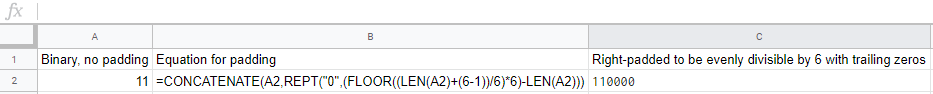### Non-ASCII characters – beware binary padding!

It was brought to my attention that my original “pure” formula was not handling foreign characters correctly. This wasn’t too surprising, but after I started digging into my own work, I was wondering why it shouldn’t work as-is; after all, the Google Sheets Unicode() function should be able to handle all non-ASCII chars, and the lookup tables should stay the same (standard ASCII) regardless of input charset. After breaking down my own function into pieces, I found the culprit – the BASE() function.

I was using BASE() to turn each Unicode (UTF-8 presumably) code point into binary, then pad to 8 digits long. Can you guess the problem? I’ll give you a hint. The standard+extended ASCII table ranges from decimal 0 to 255. If you want to use the full UTF-8 range, and map other characters, you need to go above. For example, this character – “ă”, or “latin small letter a with breve” is mapped to Unicode 259. Found the issue yet? Once you go past 255, converting from decimal (base10) to binary (base2) gives you an output that exceeds 8 bits (a byte). 259 in binary is “100000011”, which is 9 bits long. I was telling BASE() to pad all outputs to 8 bits, but when the output was already longer, instead of padding to a multiple of it, it just ignored it and left it at 9 bits long. This was breaking the rest of my formula, which relied on byte chunking (groups of 8 bits)!

Solution: There could be a solution to this, although it would be rather complicated since multiple spots in the formula rely on chunking and an expected 8 bit starting chunk.

### More resources:

I also frequently used base64 online converters, to ensure my formula work was correct, such as this instant online converter and this one.

How about you? Do you have any tips or feedback related to Base64 conversion inside Google Sheets? I didn’t cover Excel much, since I rarely ever use it, and it would take a while to come up with an Excel equivalent of my custom formula, since Excel doesn’t have functions such as REGEXREPLACE(). However, if you are interested in an Excel solution, it is probably possible to achieve using VBA – I might start with something like this as a starting point.

1/27/2021: “Pure formula” fixes

1/27/2021: Summary

Fixed issue with the “pure formula” approach. It wasn’t working with input strings that contained line breaks (e.g. `\n`), as it would trim off the first character following the line break character. This was traced back to the RegEx pattern that splits the input into individual characters – I had forgotten the flag to let the dot pattern (`.`) match all characters including line breaks!. I also took this opportunity to fix two other bugs – the handling of `'` (single quotes) and `~` (used in my formula as a delimiter)

1/27/2021: Detailed Notes
• The fix to match `.` not matching newlines was simply to add the appropriate dotall flag for RE2 (the RegEx flavor that Google Sheets uses). In effect:
• Replaced: `REGEXREPLACE(A2&"","(.{1})","\$1~")`
• With: `REGEXREPLACE(A2&"","(?s)(.{1})","\$1~")`
• In fixing the above, I noticed another issue: input strings with `'` (single quotations) were not working, period (throwing fatal error `Function UNICODE parameter 1 value should be non-empty.`)
• The fix for this was to start double escaping single quotes
• Replace: `REGEXREPLACE(A2&"","(?s)(.{1})","\$1~")`
• With: `REGEXREPLACE(REGEXREPLACE(A2&"","(?s)(.{1})","\$1~"),"'","''")`
• NOTE: Order of operations is important here! Prefixing each character with the delimiter needs to come first, or else the double escaped string (`''`) will be treated prematurely as two characters, instead of an escaped single char.
• Another issue, which had actually been bothering me from the start, is that the pure formula cannot handle input strings with `~`, since internally that is used within the formula as a delimiter.
• I finally found a solution; I was able to find a non-printable ASCII character code to use a delimiter, which should (probably) never show up in input strings!
• Replace: `SPLIT(REGEXREPLACE(REGEXREPLACE(A2&"","(?s)(.{1})","\$1~"),"'","''"),"~")`
• With: `SPLIT(REGEXREPLACE(REGEXREPLACE(A2&"","(?s)(.{1})","\$1"&CHAR(127)),"'","''"),CHAR(127))`

1.Maxime says:

Thank you. For the solution A, the encoding methods seem to work pretty good.
But i can’t get to work the decoding part. I always get some odd results which are not the decoding of the base 64 encoded input string.
You provide only the example for the encoding part in your article. Could you add an exemple of how you use the decoding functions in your google sheet please ?
(I might just be dumb. But we are 2 people facing the same problem right now ^_^ so at least I might not be dumb alone).

Have a good day !

1.joshuatz says:

I’ve updated the post based on what you noticed and reported on in your other comment; good catch and thanks for bringing to my attention! I’ve also added a new screenshot showing the usage of decoding.

2.Maxime says:

Looking at the doc https://developers.google.com/apps-script/reference/utilities/utilities it seems that base64Decode*(encoded) methods return Byte[] and not String. So the result would need to be converted to a string to be usable.

Looking at the decode method documentation provided by Google here I found an easy way to convert the byte[] to a String.
Here is the result for working base 64 decode methods :

function base64Decode(input) {
return Utilities.newBlob(Utilities.base64Decode(input)).getDataAsString();
}

function base64DecodeWebSafe(input) {
return Utilities.newBlob(Utilities.base64DecodeWebSafe(input)).getDataAsString();
}

Hope it helps.

1.joshuatz says:

Thanks for catching that Maxime! For some reason I only ever tested the encode functions (I think because at the time that is what I needed for a project) and somehow missed that it was returning byte-arrays. Your code is right on the money, and I’ve updated my post to use it and mention the edit. Thanks!

3.atom says:

“Extremly long formula” perfectly works….thanks!!!

4.John says:

This is an amazing formula! Curious how you would adjust this (if possible) so that A2 could be replaced with a range of cells… so that another arrayformula could populate all the cells in a column with the corresponding data in another column. So for example, if I placed a variation of this formula in B2 so that B2 encodes the data in A2, B3 encodes the data in A3, B4 from A4, and so on down the column range automatically when new data is added to the sheet. Possible? When I switch A2 to A2:A it gives me an error that Join can only use one range. Been futzing with this for an hour trying to get something to work.

1.joshuatz says:

Sorry for taking a while to respond. I’ve updated the post and the custom function to accept an array of cells as input. So, for your example, you could simply pass `A2:A` as the first argument to the function in cell B2 – it would automatically fill B2 and downwards with the encoded values from A2 and downwards, and would update when you added new values to the A column. Hope this helps!

5.EL says:

Joshua, great stuff! I ran into an issue with the non scripting base64 encoding vs the scripted one. If you have multiple lines in the cell that you want to be encoded it will not work correctly. The non script one will not pick up the CHAR(10) for the line break and gives the incorrect encoded result. I would love to have a non script one that makes works not sure that is possible. something like this
test:1
testing:12
result with formula = dGVzdDoxCmVzdGluZzoxMg== The result with the script = dGVzdDoxCnRlc3Rpbmc6MTI=

Not sure there is a solution but wanted to point it out.

1.joshuatz says:

Thanks for catching this! I’ve responded to you in our separate chat, but for anyone else reading this too: this issue (and two others) have just been patched in today’s (1/27/2021) update of this post!

6.EL says:

Thanks for the update!

7.Raymond says:

Great formula! Did you ever create an Excel version of your formula? Would be very useful.

8.Mike says:

Thank you joshuatz for the amazing work!
Have tried to encode to base64 the following: 00050008B470000A00000000000A00000000000A000000000000FFFFFFFF
the non-scripted version gives: MDAwNTAwMDhCNDcwMDAwQTAwMDAwMDAwMDAwQTAwMDAwMDAwMDAwQTAwMDAwMDAwMDAwMEZGRkZGRkZG
1.joshuatz says: